﻿ Cat Writes An Equation On The Fence Stock Image - Image of write, math: 147687885

# Cat writes an equation on the fence.

## The cat writes a funny math equation in chalk on the wooden fence

More similar stock imagesThe teacher writes on a board with chalk a formula for electric current.Charming man writes with chalk.TeacherChemist at the chalkboardMan`s hand writing equation on the notebook sheet in a cageHappy girl student, the teacher writes on blackboard chalk formMan writes mathematical equations on whiteboardSuccessful scientist writes a formula on a glass BoardScientist writes formula planning to invent new fertilizer, theoretical research
More stock photos from Iryna Kuznetsova's portfolioCat in glasses near a fence 2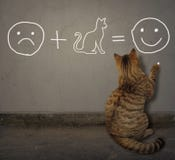Cat writes an equation on the wall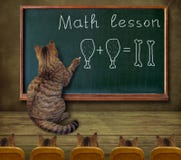Cat teaches math to students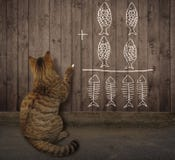Cat writes a math equation on a fence 2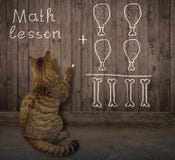Cat writes a math equation on a fenceCat in glasses near a fence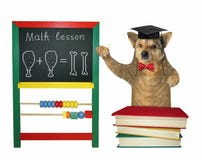Dog teaches near a blackboardCat writes a funny math equation on a fence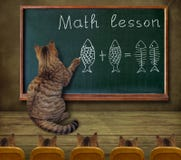Cat teaches math to students 2
Related categories